# Number world.ppt

28 May 2023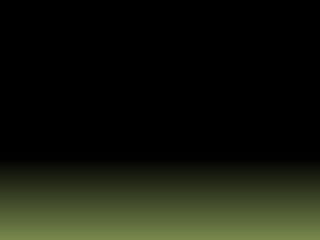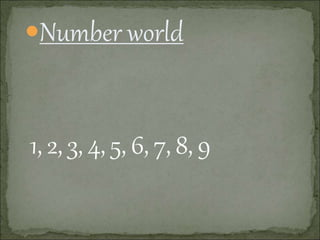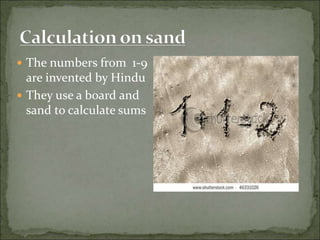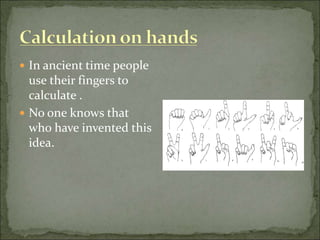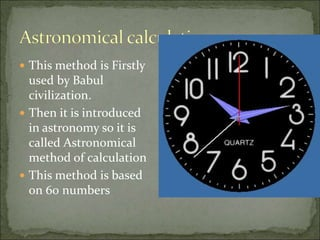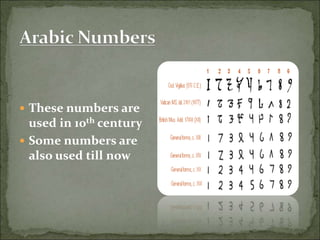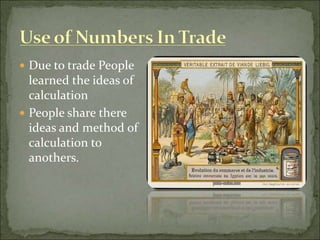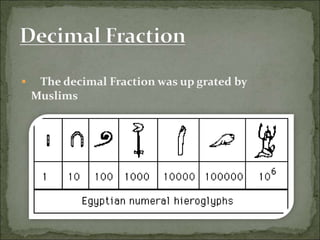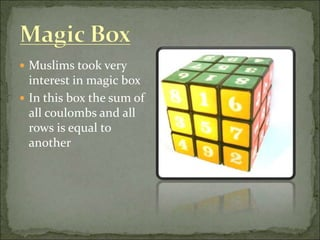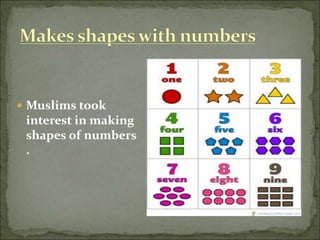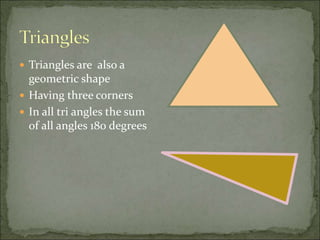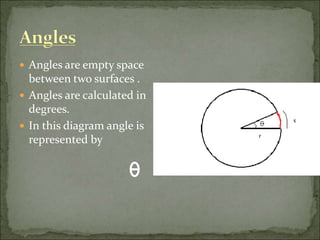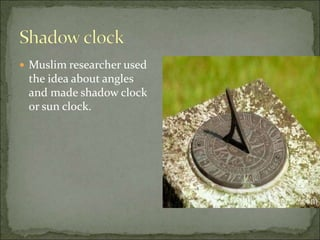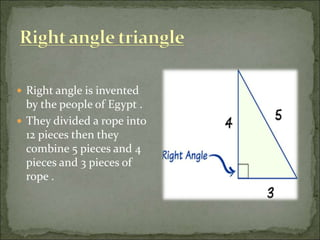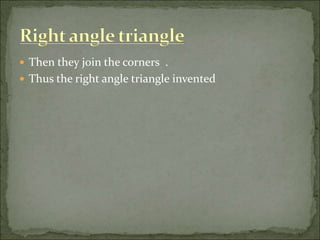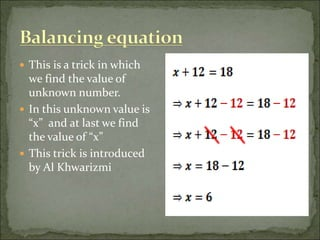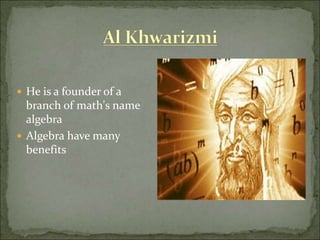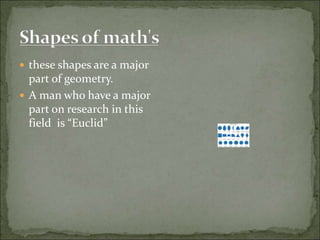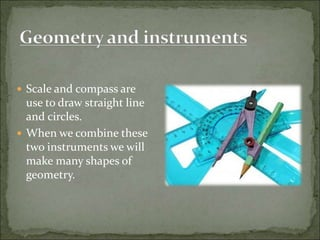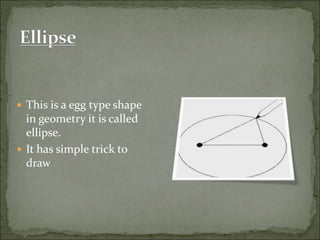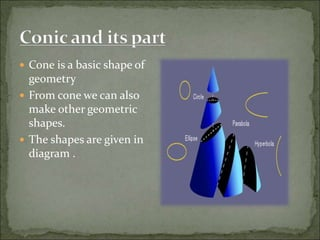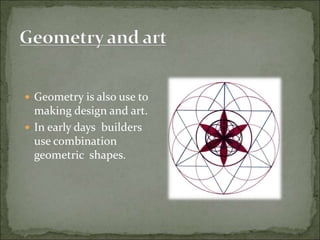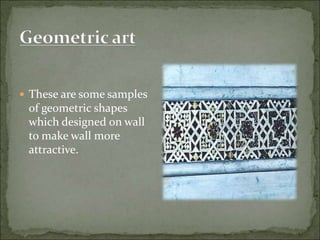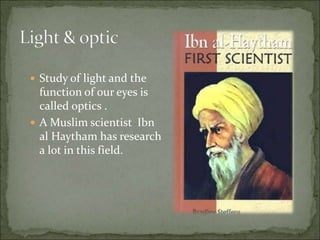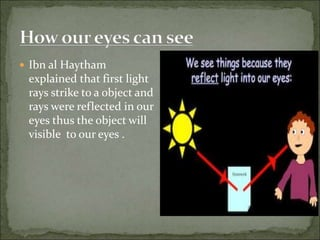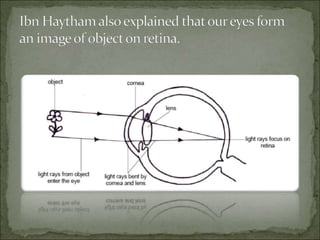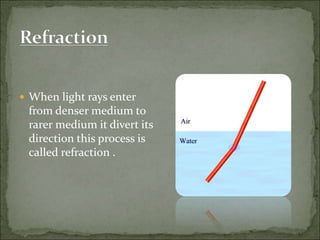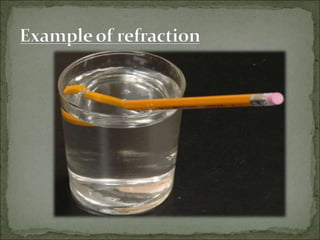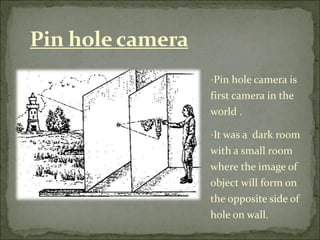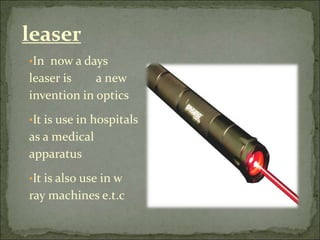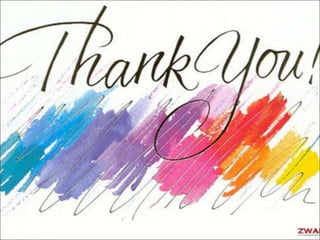1 sur 31

### Number world.ppt

• 2. Number world
• 3.  The numbers from 1-9 are invented by Hindu  They use a board and sand to calculate sums
• 4.  In ancient time people use their fingers to calculate .  No one knows that who have invented this idea.
• 5.  This method is Firstly used by Babul civilization.  Then it is introduced in astronomy so it is called Astronomical method of calculation  This method is based on 60 numbers
• 6.  These numbers are used in 10th century  Some numbers are also used till now
• 7.  Due to trade People learned the ideas of calculation  People share there ideas and method of calculation to anothers.
• 8.  The decimal Fraction was up grated by Muslims
• 9.  Muslims took very interest in magic box  In this box the sum of all coulombs and all rows is equal to another
• 10.  Muslims took interest in making shapes of numbers .
• 11.  Triangles are also a geometric shape  Having three corners  In all tri angles the sum of all angles 180 degrees
• 12.  Angles are empty space between two surfaces .  Angles are calculated in degrees.  In this diagram angle is represented by
• 13.  Muslim researcher used the idea about angles and made shadow clock or sun clock.
• 14.  Right angle is invented by the people of Egypt .  They divided a rope into 12 pieces then they combine 5 pieces and 4 pieces and 3 pieces of rope .
• 15.  Then they join the corners .  Thus the right angle triangle invented
• 16.  This is a trick in which we find the value of unknown number.  In this unknown value is “x” and at last we find the value of “x”  This trick is introduced by Al Khwarizmi
• 17.  He is a founder of a branch of math's name algebra  Algebra have many benefits
• 18.  these shapes are a major part of geometry.  A man who have a major part on research in this field is “Euclid”
• 19.  Scale and compass are use to draw straight line and circles.  When we combine these two instruments we will make many shapes of geometry.
• 20.  This is a egg type shape in geometry it is called ellipse.  It has simple trick to draw
• 21.  Cone is a basic shape of geometry  From cone we can also make other geometric shapes.  The shapes are given in diagram .
• 22.  Geometry is also use to making design and art.  In early days builders use combination geometric shapes.
• 23.  These are some samples of geometric shapes which designed on wall to make wall more attractive.
• 24.  Study of light and the function of our eyes is called optics .  A Muslim scientist Ibn al Haytham has research a lot in this field.
• 25.  Ibn al Haytham explained that first light rays strike to a object and rays were reflected in our eyes thus the object will visible to our eyes .
• 27.  When light rays enter from denser medium to rarer medium it divert its direction this process is called refraction .
• 29. •Pin hole camera is first camera in the world . •It was a dark room with a small room where the image of object will form on the opposite side of hole on wall. Pin hole camera
• 30. •In now a days leaser is a new invention in optics •It is use in hospitals as a medical apparatus •It is also use in w ray machines e.t.c leaser